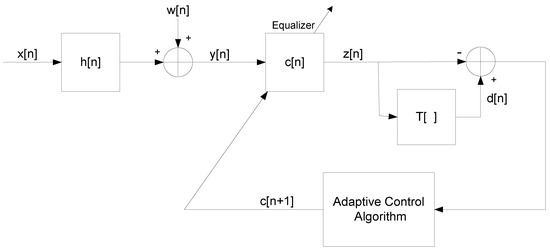Next Article in Journal
Negation of Belief Function Based on the Total Uncertainty Measure
Next Article in Special Issue
Quaternion Entropy for Analysis of Gait Data
Previous Article in Journal
Acknowledgement to Reviewers of Entropy in 2018
Previous Article in Special Issue
SINR- and MI-Based Maximin Robust Waveform Design
Open AccessArticle

# A New Efficient Expression for the Conditional Expectation of the Blind Adaptive Deconvolution Problem Valid for the Entire Range ofSignal-to-Noise Ratio

Department of Electrical and Electronic Engineering, Ariel University, Ariel 40700, Israel
Entropy 2019, 21(1), 72; https://doi.org/10.3390/e21010072
Received: 10 December 2018 / Revised: 9 January 2019 / Accepted: 14 January 2019 / Published: 15 January 2019
(This article belongs to the Special Issue Information Theory Applications in Signal Processing)
In the literature, we can find several blind adaptive deconvolution algorithms based on closed-form approximated expressions for the conditional expectation (the expectation of the source input given the equalized or deconvolutional output), involving the maximum entropy density approximation technique. The main drawback of these algorithms is the heavy computational burden involved in calculating the expression for the conditional expectation. In addition, none of these techniques are applicable for signal-to-noise ratios lower than 7 dB. In this paper, I propose a new closed-form approximated expression for the conditional expectation based on a previously obtained expression where the equalized output probability density function is calculated via the approximated input probability density function which itself is approximated with the maximum entropy density approximation technique. This newly proposed expression has a reduced computational burden compared with the previously obtained expressions for the conditional expectation based on the maximum entropy approximation technique. The simulation results indicate that the newly proposed algorithm with the newly proposed Lagrange multipliers is suitable for signal-to-noise ratio values down to 0 dB and has an improved equalization performance from the residual inter-symbol-interference point of view compared to the previously obtained algorithms based on the conditional expectation obtained via the maximum entropy technique. View Full-Text
Show FiguresFigure 1

MDPI and ACS Style

Pinchas, M. A New Efficient Expression for the Conditional Expectation of the Blind Adaptive Deconvolution Problem Valid for the Entire Range ofSignal-to-Noise Ratio. Entropy 2019, 21, 72.

Show more citation formats Show less citations formats
Note that from the first issue of 2016, MDPI journals use article numbers instead of page numbers. See further details here.

1